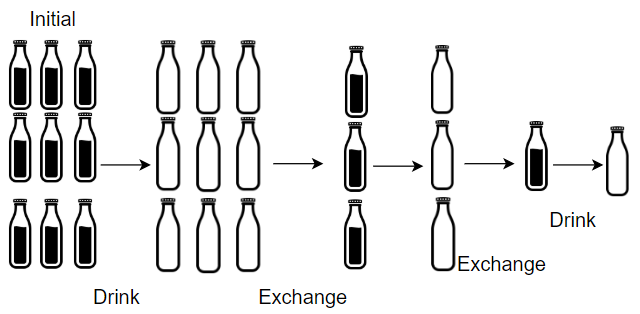# 1518. Water Bottles

Easy
There are `numBottles` water bottles that are initially full of water. You can exchange `numExchange` empty water bottles from the market with one full water bottle.
The operation of drinking a full water bottle turns it into an empty bottle.
Given the two integers `numBottles` and `numExchange`, return the maximum number of water bottles you can drink.
Example 1:Input: numBottles = 9, numExchange = 3
Output:
13
Explanation:
You can exchange 3 empty bottles to get 1 full water bottle.
Number of water bottles you can drink: 9 + 3 + 1 = 13.
Example 2:Input: numBottles = 15, numExchange = 4
Output:
19
Explanation:
You can exchange 4 empty bottles to get 1 full water bottle.
Number of water bottles you can drink: 15 + 3 + 1 = 19.
Constraints:
• `1 <= numBottles <= 100`
• `2 <= numExchange <= 100`

### 解題

Runtime: 0 ms, faster than 100.00%
Memory Usage: 1.9 MB, less than 97.96%
func numWaterBottles(numBottles int, numExchange int) int {
ans := 0
empty := 0
for numBottles>0 || empty >= numExchange {
ans += numBottles
empty += numBottles
numBottles = empty/numExchange
empty = empty%numExchange
}
return ans
}